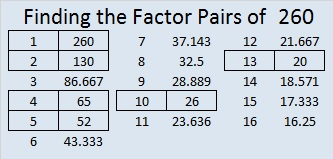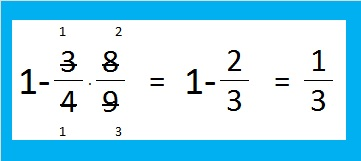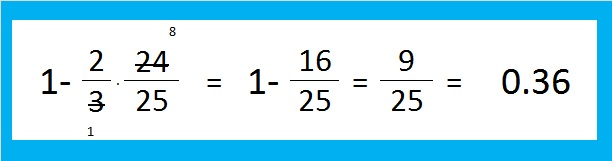# 260 Some Thoughts on Those Four Consecutive Numbers

• 260 is a composite number.
• Prime factorization: 260 = 2 x 2 x 5 x 13, which can be written 260 = (2^2) x 5 x 13
• The exponents in the prime factorization are 2, 1 and 1. Adding one to each and multiplying we get (2 + 1)(1 + 1)(1 + 1) = 3 x 2 x 2 = 12. Therefore 260 has 12 factors.
• Factors of 260: 1, 2, 4, 5, 10, 13, 20, 26, 52, 65, 130, 260
• Factor pairs: 260 = 1 x 260, 2 x 130, 4 x 65, 5 x 52, 10 x 26, or 13 x 20
• Taking the factor pair with the largest square number factor, we get √260 = (√4)(√65) = 2√65 ≈ 16.125Recently I wrote about the smallest four-consecutive-numbers whose square roots could all be simplified. The same numbers were also the smallest four consecutive numbers to have the same number of factors.Each of those numbers had 6 factors, and guess what, ANY number with exactly 6 factors can have its square root simplified. The prime factorization of ANY number with exactly 6 factors can be expressed in one of the three following ways:Since numbers with six factors always have a prime factor raised to a power greater than one, they can always have their square roots simplified. The fact that those four consecutive numbers have the same number of factors makes them extraordinary; that they all can have their square roots simplified is merely the natural consequence of that extraordinary fact.

# 252 How likely can this square root be simplified?

• 252 is a composite number.
• Prime factorization: 252 = 2 x 2 x 3 x 3 x 7, which can be written 252 = (2^2) x (3^2) x 7
• The exponents in the prime factorization are 2, 2, and 1. Adding one to each and multiplying we get (2 + 1)(2 + 1)(1 + 1) = 3 x 3 x 2 = 18. Therefore 252 has 18 factors.
• Factors of 252: 1, 2, 3, 4, 6, 7, 9, 12, 14, 18, 21, 28, 36, 42, 63, 84, 126, 252
• Factor pairs: 252 = 1 x 252, 2 x 126, 3 x 84, 4 x 63, 6 x 42, 7 x 36, 9 x 28, 12 x 21, or 14 x 18
• Taking the factor pair with the largest square number factor, we get √252 = (√7)(√36) = 6√7 ≈ 15.875The square root of a whole number can be simplified if it has a square number factor. How likely is that condition met by any random whole number?

4 is 2 x 2 and therefore a square number.  1 out of every four whole numbers (or 25%) is divisible by 4

3^2 = 9. Likewise 1 out of every nine whole numbers is divisible by square number 9 (about 11.1%).

Some numbers, like 252, are divisible by both 4 and 9. (1 out of every 36 numbers are divisible by both 4 and 9.)Thus 1/3 of all whole numbers are divisible by 4, 9 or both.

That means that 2/3 of the numbers in the set of all whole numbers are NOT divisible by 4, 9 or both. It is often easier to compute the probability of something NOT happening and then subtract that fraction from 1 to determine the probability of something actually happening. The probability a number is NOT divisible by 4 is 3/4 while the probability a whole number is NOT divisible by 9 is 8/9. We get the same result either way.1/3 of all whole numbers (about 33.3%) are divisible by either 4 or 9! That fact is very cool because it is so easy to tell if a number is divisible by 4 or 9: If the last 2 digits of a number is divisible by 4, the entire number is divisible by 4 and if the sum of the digits of a whole number is divisible by 9, that whole number is divisible by 9.

It is also very easy to tell if a number is divisible by 5 x 5 or 25. If the last two digits of the number are 25, 50, 75 or 00, then it is divisible by 25. Let’s compute how likely it is that the square root of a number can be simplified because that number is divisible by 4, 9, or 25.Thus 36% of all whole numbers are divisible by 4, 9, or 25 and therefore have square roots that can be simplified! It is not as easy to tell if a number is divisible by 49, 121, 169, or any other number that is the perfect square of a prime number. The percentage of numbers that are divisible by these other perfect squares doesn’t go up much more either. Consider this infinite product subtracted from 1:When I’ve computed the partial product up to 3480/(59 x 59) and subtracted it from 1, the probability only increased to 39.010%. I used excel to compute the probability of a number being divisible by a square factor up to 1,495,729 (which is 1223^2) and it is only 39.201%. There isn’t much change in the percentage between the 17th prime number (59) and the 200th prime number (1223).

As n gets larger (n^2 -1)/(n^2) gets closer and closer to 1. I conclude that the probability that a random whole number can have its square root simplified is about 40%.

# 245 – The Last of Four Consecutive Numbers

• 245 is a composite number.
• Prime factorization: 245 = 5 x 7 x 7, which can be written 245 = 5 x (7^2)
• The exponents in the prime factorization are 1 and 2. Adding one  to each and multiplying we get (1 + 1)(2 + 1) = 2 x 3 = 6. Therefore 245 has 6 factors.
• Factors of 245: 1, 5, 7, 35, 49, 245
• Factor pairs: 245 = 1 x 245, 5 x 49, or 7 x 35
• Taking the factor pair with the largest square number factor, we get √245 = (√5)(√49) = 7√5 ≈ 15.652I was surprised when I noticed that the square roots of these 4 consecutive numbers – 242, 243, 244, and 245 could all be simplified.

The square root of a whole number can only be simplified if that whole number has a square number as one of its factors. All four of these numbers meet that condition, and they are the first four consecutive numbers to do so.

For numbers less than or equal to 240, there are only 3 sets of 3 consecutive square roots that can be simplified.

• √48 = 4√3
• √49 = 7
• √50 = 5√2
• √98 = 7√2
• √99 = 3√11
• √100 = 10
• √124 = 2√31
• √125 = 5√5
• √126 = 3√14

242, 243, 244, and 245 also have another distinction. They each have exactly 6 factors and are the smallest consecutive four numbers to have the same number of factors.